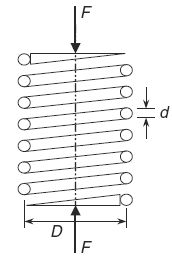Related Resources: calculators

### Helical Compression Spring Critical Frequency Critical Frequency Formula and Calculator

Helical Compression Spring Critical Frequency Formula and Calculator

Helical springs, such as those used in the valve trains of internal combustion engines, can fail if the frequency of loading coincides with the natural, or critical, frequency of the spring, called resonance. Different end-conditions, like those summarized in Table 1, produce different critical frequencies. To avoid problems, it is usually recommended that the spring design be such that its critical frequency is 15 to 20 times the frequency of the applied cyclic loading frequency.Helical Compression Spring

For a helical spring positioned between flat parallel surfaces, where one of the surfaces is driven by a sinusoidal forcing function, the critical frequency ( f cr) in cycles per second (Hz) is given by the following formula:

Eq 1
f cr = 0.5 ( k / m )0.5

The mass (m) can be found by multiplying the density (ρ) of the spring material times its volume. The development of an expression for the mass of the active part of a spring is given by the following formula:

Eq. 2
m = density · volume

m = ρ A l m

Where:

A = π d2 / 4
l = π D Na

m = ρ π d2 D Na / 4

Including the expression for the the number of active coils ( Na ) into mass formula:

Na = ( d4 G ) / ( 8 D3 k )

thus:

Eq. 3
m = ( ρ π d6 G ) / ( 32 D2 k )

Substitute the expression for the mass (m) Eq. 3 into expression for critical frequency ( f cr) Eq. 1:

Eq 4
( f cr) = ( D K ) / ( π d3 ) [ 8 / ( ρ G ) ]0.5

As stated earlier, multiply the critical frequency by 15 to 20 to avoid resonance with the frequency of the cyclic loading on the spring.

End-Condition Constant (α) Helical Springs - Table 1

 α End Condition 0.5 Both ends supported on flat parallel surfaces 0.7 One end supported on flat surface, other end hinged 1 Both ends hinged 2 One end support on flat surface, other end free

Where:

f cr = Critical frequency (Hz),
m = mass of active pat of spring,
k = spring constant (lb/in, N/m),
ρ = density (slug/ft3, kg/m3),
Na = number of active coils in spring,
d = diameter of spring wire (in, m),
D = mean diameter of coil helical spring (in, m),
G = shear modulus of elasticity (lbs/in2, N/m2),
FOS = Spring multiplier applied to cyclic loading frequency (15 to 20).

Related:

Source:

Mark's Calculations for Machine Design
Thomas H. Brown, Jr.
Faculty Associate
Institute for Transportation Research and Education
NC State University
Raleigh, North Carolina